Function Repository Resource:

# LinearAlgebraMod

Perform matrix operations over a finite field

Contributed by: Daniel Lichtblau
 ResourceFunction["LinearAlgebraMod"][op,mat,p] performs the operation op on matrix mat using prime modulus p. ResourceFunction["LinearAlgebraMod"][LinearSolve,mat,rhs,p] solves mat.x ==rhs for x, modulo p. ResourceFunction["LinearAlgebraMod"][CharacteristicPolynomial,mat,t,p] computes the characteristic polynomial for square matrix mat using t as the polynomial variable. ResourceFunction["LinearAlgebraMod"][op] represents an operator form that can be applied to matrix and modulus arguments. ResourceFunction["LinearAlgebraMod"][op,p] represents an operator form, with the operation and modulus both specified, that can be applied to a matrix.

## Details and Options

When LinearSolve is the operation, a vector or second matrix must be given as the right-hand side.
The operation names can be given either as symbols or strings.
The modulus is 0 by default.
ResourceFunction["LinearAlgebraMod"] accepts an Extension option. It must be a univariate polynomial that is irreducible modulo the prime p, or irreducible over the rationals if the modulus is 0.
ResourceFunction["LinearAlgebraMod"] performs linear algebra over a finite field or an algebraic extension of the rationals, with an input matrix comprised of (not necessarily univariate) polynomial functions.

## Examples

### Basic Examples (11)

Start with a 2×3 polynomial matrix in two variables, one of which will be used as an algebraic extension:

 In:=The polynomial defining the extension:

 In:=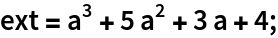Reduce to row echelon form over the finite extension field of integers mod 7 with 73=343 elements, given as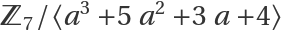:

 In:=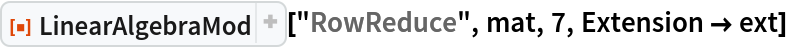Out=Compute the inverse of the submatrix comprised of the first two columns:

 In:=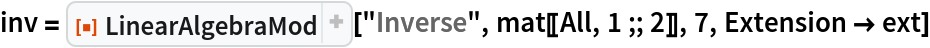Out=Check that this inverse multiplied by mat gives the 2×2 identity matrix:

 In:=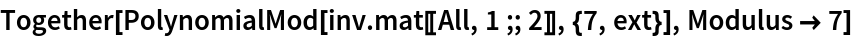Out=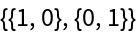Use the last column as a right-hand side for a matrix equation:

 In:=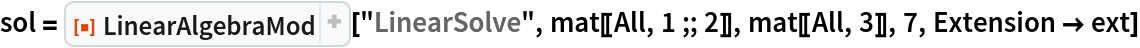Out=Check the result:

 In:=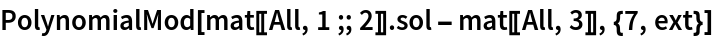Out=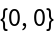Find a basis for the null space of the full matrix:

 In:=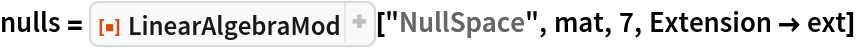Out=Check that it has only null vectors for mat:

 In:=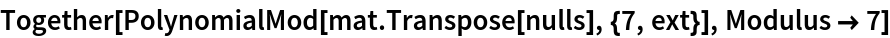Out=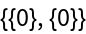Since there are three columns and the null space is generated by one vector, the rank must be 2:

 In:=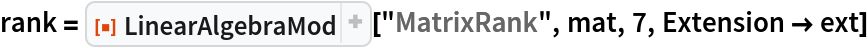Out=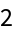Compute the characteristic polynomial for the matrix augmented by a row of ones:

 In:=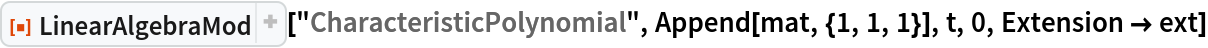Out=### Scope (2)

One need not use an extension field:

 In:=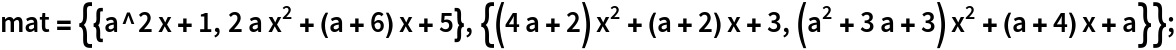In:=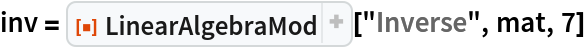Out=Verify the inverse:

 In:=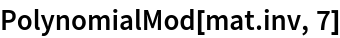Out=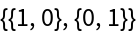Apart from cancellation of factors, the denominators in the inverse will be constant multiples of the matrix determinant:

 In:=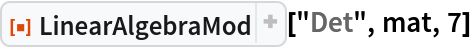Out=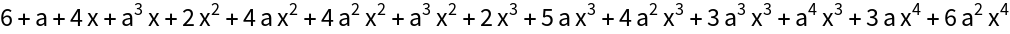Use an operator form of LinearAlgebraMod:

 In:=In:=Out=Check the solution:

 In:=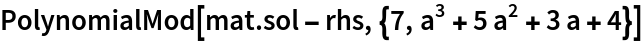Out=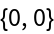Use an operator form of LinearAlgebraMod with operation and modulus both specified:

 In:=Out=Use a matrix for the right-hand side:

 In:=Out=Check that first column agrees with the previous solution:

 In:=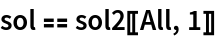Out=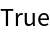Check that the result is correct:

 In:=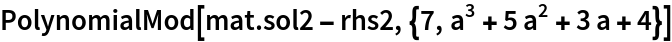Out=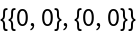### Properties and Relations (2)

Define a matrix of integers:

 In:=Out=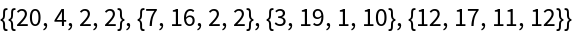For integer matrices, operations supported by LinearAlgebraMod are equivalent to their built-in counterparts using the Modulus option:

 In:=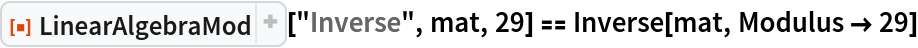Out=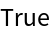### Possible Issues (2)

Symbolic linear algebra can suffer from expression swell (the phenomenon wherein outputs and/or intermediate computations get much larger than inputs).

Define a random polynomial matrix generator:

 In:=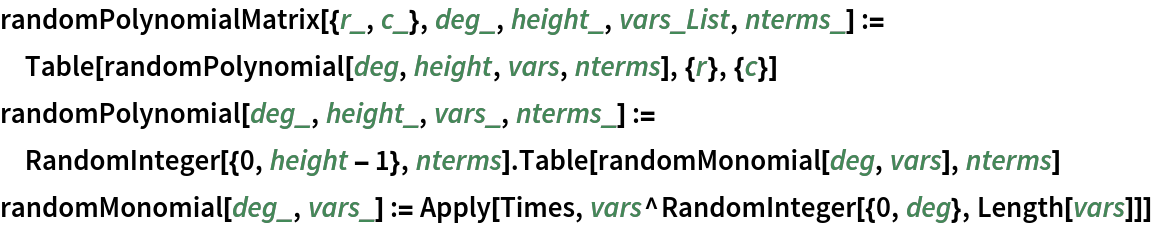Create a random 3×3 matrix of polynomials in two variables with up to seven terms having coefficients between 0 and 70, each of which can have a degree up to 4:

 In:=Solve the system:

 In:=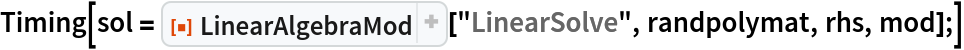Out=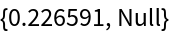Check the result:

 In:=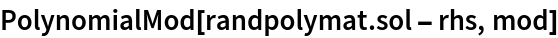Out=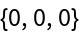Note the difference in size between input and result:

 In:=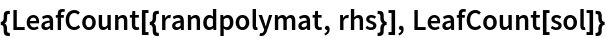Out=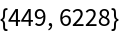Create a 6×6 example:

 In:=This takes significantly longer:

 In:=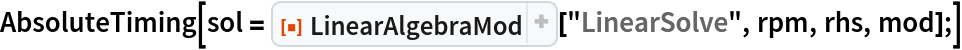Out=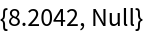The factor between output and input sizes has also grown:

 In:=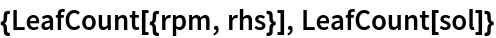Out=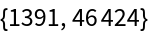Also note that using the built-in LinearSolve for the non-modular case is also on the slow side, though faster than LinearAlgebraMod:

 In:=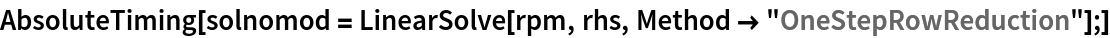Out=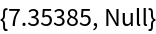It produces a similarly large result:

 In:=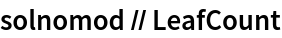Out=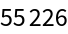The determinant can be found somewhat faster:

 In:=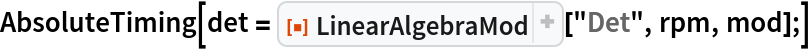Out=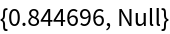In:=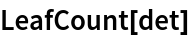Out=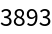## Version History

• 7.0.1 – 05 December 2022
• 7.0.0 – 01 September 2021